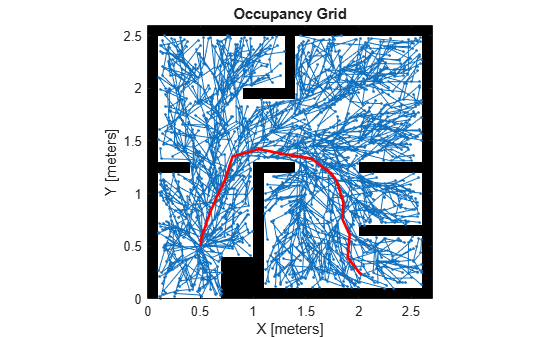# plannerRRTStar

Create an optimal RRT path planner (RRT*)

## Description

The `plannerRRTStar` object creates an asymptotically-optimal RRT planner, RRT*. The RRT* algorithm converges to an optimal solution in terms of the state space distance. Also, its runtime is a constant factor of the runtime of the RRT algorithm. RRT* is used to solve geometric planning problems. A geometric planning problem requires that any two random states drawn from the state space can be connected.

## Creation

### Syntax

``planner = plannerRRTStar(stateSpace,stateVal)``

### Description

example

````planner = plannerRRTStar(stateSpace,stateVal)` creates an RRT* planner from a state space object, `stateSpace`, and a state validator object, `stateVal`. The state space of `stateVal` must be the same as `stateSpace`. `stateSpace` and `stateVal` also sets the `StateSpace` and `StateValidator` properties of the `planner` object. ```

## Properties

expand all

Constant used to estimate the near neighbors search radius, specified as a positive scalar. With a larger ball radius, the searching radius reduces slower as the number of nodes in the tree increases. The radius is estimated as following:

`$r=\mathrm{min}\left({\left\{\frac{\gamma \mathrm{ln}\left(n\right)}{n{V}_{d}}\right\}}^{1/d},\eta \right)$`

where:

• d — Dimension of the state space

• n — Number of nodes in the search tree

• η — The value of the `MaxConnectionDistance` property

• Vd — Volume of the unit ball in the dth dimension

Data Types: `single` | `double`

Decide if the planner continues to optimize after the goal is reached, specified as `false` or `true`. The planner also terminates regardless of the value of this property if the maximum number of iterations or maximum number of tree nodes is reached.

Data Types: `logical`

State space for the planner, specified as a state space object. You can use state space objects such as `stateSpaceSE2`, `stateSpaceDubins`, and `stateSpaceReedsShepp`. You can also customize a state space object using the `nav.StateSpace` object.

State validator for the planner, specified as a state validator object. You can use state validator objects such as `validatorOccupancyMap` and `validatorVehicleCostmap`.

Maximum number of nodes in the search tree (excluding the root node), specified as a positive integer.

Data Types: `single` | `double`

Maximum number of iterations, specified as a positive integer.

Data Types: `single` | `double`

Maximum length of a motion allowed in the tree, specified as a scalar.

Data Types: `single` | `double`

Callback function to determine whether the goal is reached, specified as a function handle. You can create your own goal reached function. The function must follow this syntax:

` function isReached = myGoalReachedFcn(planner,currentState,goalState)`

where:

• `planner` — The created planner object, specified as `plannerRRTStar` object.

• `currentState` — The current state, specified as a three element real vector.

• `goalState` — The goal state, specified as a three element real vector.

• `isReached` — A boolean variable to indicate whether the current state has reached the goal state, returned as `true` or `false`.

To use custom `GoalReachedFcn` in code generation workflow, this property must be set to a custom function handle before calling the plan function and it cannot be changed after initialization.

Data Types: `function handle`

Probability of choosing the goal state during state sampling, specified as a real scalar in [0,1]. The property defines the probability of choosing the actual goal state during the process of randomly selecting states from the state space. You can start by setting the probability to a small value such as `0.05`.

Data Types: `single` | `double`

## Object Functions

 `plan` Plan path between two states `copy` Create copy of planner object

## Examples

collapse all

Create a state space.

`ss = stateSpaceSE2;`

Create a `occupancyMap`-based state validator using the created state space.

`sv = validatorOccupancyMap(ss);`

Create an occupany map from an example map and set map resolution as 10 cells/meter.

```load exampleMaps.mat map = occupancyMap(simpleMap, 10); sv.Map = map;```

Set validation distance for the validator.

`sv.ValidationDistance = 0.01;`

Update state space bounds to be the same as map limits.

`ss.StateBounds = [map.XWorldLimits; map.YWorldLimits; [-pi pi]];`

Create RRT* path planner and allow further optimization after goal is reached.

```planner = plannerRRTStar(ss,sv); planner.ContinueAfterGoalReached = true;```

Reduce max iterations and increase max connection distance.

```planner.MaxIterations = 2500; planner.MaxConnectionDistance = 0.3;```

Set the start and goal states.

```start = [0.5, 0.5 0]; goal = [2.5, 0.2, 0];```

Plan a path with default settings.

```rng(100, 'twister') % repeatable result [pthObj, solnInfo] = plan(planner,start,goal);```

Visualize the results.

```map.show; hold on; plot(solnInfo.TreeData(:,1),solnInfo.TreeData(:,2), '.-'); % tree expansion plot(pthObj.States(:,1),pthObj.States(:,2),'r-','LineWidth',2); % draw path```Karaman, S. and E. Frazzoli. "Sampling-Based Algorithms for Optimal Motion Planning." The International Journal of Robotics Research. Vol. 30, Number 7, 2011, pp 846 – 894.